# Random event

(diff) ← Older revision | Latest revision (diff) | Newer revision → (diff)

event

Any combination of outcomes of an experiment that has a definite probability of occurrence.

Example 1. In the throwing of two dice, each of the 36 outcomes can be represented as a pair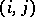, whereis the number of dots on the upper face of the first dice andthe number on the second. The event "the sum of the dots is equal to 11" is just the combination of the two outcomes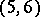and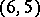.

Example 2. In the random throwing of two points into an interval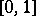, the set of all outcomes can be represented as the set of points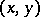(whereis the value of the first point andthat of the second) in the square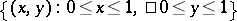. The event "the length of the interval joining x and y is less than a, 0<a< 1" is just the set of points in the square whose distance from the diagonal passing through the origin is less than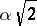.

Within the limits of the generally accepted axiomatics of probability theory (see ), where at the base of the probability model lies a probability space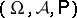(is a space of elementary events, i.e. the set of all possible outcomes of a given experiment,is a-algebra of subsets ofand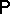is a probability measure defined on), random events are just the sets which belong to.

In the first of the above examples,is a finite set of 36 elements: the pairs,;is the class of all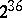subsets of(includingitself and the empty set), and for every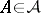the probability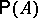is equal to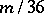, whereis the number of elements of. In the second example,is the set of points in the unit square,is the class of its Borel subsets andis ordinary Lebesgue measure on(which for simple figures coincides with their area).

The classof events associated withforms a Boolean ring with identity with respect to the operations(symmetric difference) and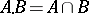(it has a multiplicative identity), that is, it forms a Boolean algebra. The functiondefined on this Boolean algebra has all the properties of a norm except one: it does not follow fromthat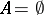. By declaring two events to be equivalent if the-measure of their symmetric difference is zero, and considering equivalence classesinstead of events, one obtains the normalized Boolean algebra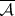of classes. This observation leads to another possible approach to the axiomatics of probability theory, in which the basic object is not the probability space connected with a given experiment, but a normalized Boolean algebra of random events (see , ).